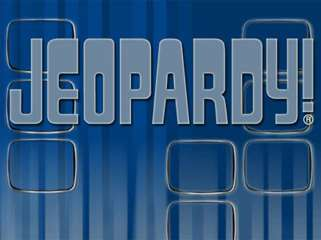Download PresentationCRCT Math Jeopardy 1

# CRCT Math Jeopardy 1

Download Presentation## CRCT Math Jeopardy 1

- - - - - - - - - - - - - - - - - - - - - - - - - - - E N D - - - - - - - - - - - - - - - - - - - - - - - - - - -
##### Presentation Transcript

1. Let’s Play 3rd Grade Algebra and Data

2. Patterns

3. Unknowns

4. Formulas

5. Charts and Tables

6. Graphs

7. Analyzing Data

8. Patterns Unknowns Formulas Charts and Tables Graphs Analyzing Data • 10 • 10 • 10 • 10 • 10 • 10 20 20 20 20 20 20 30 30 30 30 30 30 40 40 40 40 40 40 50 50 50 50 50 50

9. Final Jeopardy

11. Students who will succeed on the CRCT

12. Who are PCES Third Graders?

13. Which number is missing from this pattern? • 41, 44, 47, ___, 53, 56 • 50 • 48 • 52 • 51

14. A. 50

15. Which figure will be the 10th figure in this pattern?

16. B.

17. Which number comes next in this pattern? • 85, 81, 77, 73, 69, 65, 61, ___ • 51 • 59 • 60 • 57

18. D. 57

19. Which best describes this pattern? • 4, 8, 12, 16, 20, 24, 28, 32 • Multiply by 2 to get the next number • Add 4 to get the next number • Multiply by 3 to get the next number • Subtract 4 to get the next number

20. B. Add 4 to get the next number

21. Which best describes this pattern? • Add 1 more side to get the next figure • Hexagon will be the next figure. • Double the number of sides each time • There is no pattern.

22. A. Add 1 more side to get the next figure

23. What number goes in the to make the number sentence TRUE? 4 x = 32 A. 7 B. 8 C. 9 D. 6

24. B. 8

25. What is the missing number? 28 ÷= 4 A. 5 B. 6 C. 7 D. 8

26. C. 7

27. Which number belongs in the to make the number sentence TRUE ? 35 + = 24 + 35 A. 0 B. 59 C. 35 D. 24

28. D. 24

29. Which number belongs in the to make the number sentence TRUE? 45 + = 65 + 35 A. 55 B. 65 C. 75 D. 85

30. A. 55

31. What is the missing number? • 8 x 3 = 4 x ___ • A. 3 • 7 • 6 • 8

32. C. 6

33. Look at the rectangle below. Jim added 2 + 4 + 2 + 4 = 12. What did he find using this number sentence? A. The perimeter of the rectangle B. The area of the rectangle C. The area of a square D. The area of a rectangle 4 cm 2 cm 2 cm 4 cm

34. A. The perimeter of the rectangle

35. Look at the square. Dan added • 3 + 3 + 3 + 3 = 12. What did he find? • The area of the square. • The perimeter of the square. • The area of a rectangle. • The area of a triangle. 3 ft. 3 ft. 3 ft. 3 ft.

36. B. The perimeter of the square.

37. 4 cm 2 cm 2 cm 4 cm • Look at the rectangle below. Pam multiplied 4 x 2 = 8. Why did she do that? • She was finding the area of the rectangle. • She was finding the perimeter of the rectangle. • She was finding the length of 2 sides. • To find perimeter, you multiply the sides.

38. A. She was finding the area of the rectangle.

39. Al found the perimeter of the square below by adding 5 + 5 + 5 + 5 = 20 cm. Which would be another way to find the perimeter of the square? • 4 + 4 + 4 + 4 + 4 = 20 cm • 5 x 5 = 25 square cm • 4 x 5 = 20 cm • 4 x 4 = 16 square cm 5 cm 5 cm 5 cm 5 cm

40. C. 4 x 5 = 20 because multiplying by 4 is the same as adding 5 four times

41. Look at the square below. Jane multiplied 5 x 5 = 25. What was she trying to find? A. The perimeter of the square in cm. B. The area of the square in square cm. C. The distance around the square in cm. D. The distance from one side of the square to the other in cm. 5 cm 5 cm 5 cm 5 cm

42. B. The area of the square in square cm.

43. Look at the table about favorite fruits. • How many more liked apples best than pears? • 1 • 2 • 3 • 4

44. B. 2

45. Look at the tally chart about favorite fruits. How many students like pears or peaches? A. 11 B. 1 C. 9 D. 8

46. A. 11

47. Look at the table of favorite colors. If a bar graph is created, which color will have the tallest bar? • A. Green • Orange • Blue • Red

48. C. Blue